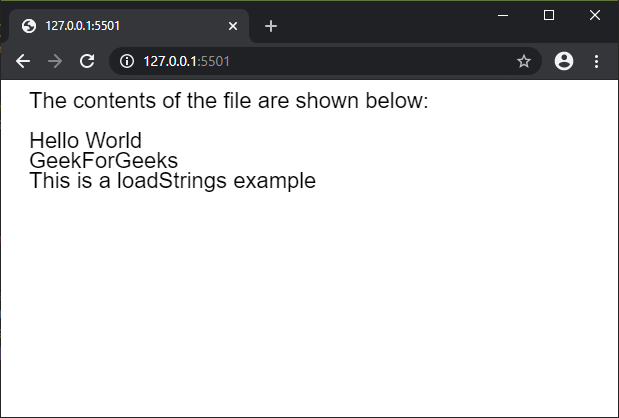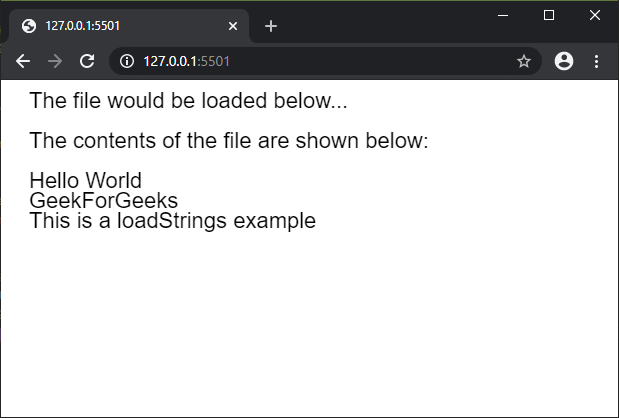# p5.js | loadStrings() Function

• Last Updated : 13 Mar, 2020

The loadStrings() function is used to read the contents of a file and create an array of strings with each of the lines of the file. The file to be read must be located at the sketch directory if the file name is used, otherwise, a URL to the file can be specified.

This function is recommended to be called in the preload() function to ensure that the function is executed before the other functions.

Syntax:

`loadStrings( filename, callback, errorCallback )`

Parameters: This function accept three parameter as mentioned above and described below:

• filename: This is a string which denotes the name of the file or the url to load the file from.
• callback: This is a function which is called after the function is successfully executed. The first argument for this function is the strings array.
• errorCallback: This is a function which is called if there is any error in executing the function. The first argument for this function is the error response.

Below examples illustrate the loadStrings() function in p5.js:

Example 1:

 `let result; ` `  `  `function` `preload() { ` `    ``result = loadStrings(``"test_file.txt"``); ` `} ` `  `  `function` `setup() { ` `    ``createCanvas(600, 300); ` `    ``textSize(22); ` `} ` `  `  `function` `draw() { ` `    ``clear(); ` `    ``text(``"The contents of the file "` `        ``+ ``"are shown below:"``, 20, 20); ` `  `  `    ``// Check if the strings array ` `    ``// is non-empty before displaying ` `    ``// the contents ` `    ``if` `(result.length > 0) { ` `        ``for` `(let i = 0; i < result.length; i++) { ` `            ``text(result[i], 20, 60 + i * 20); ` `        ``} ` `    ``} ` `    ``else` `{ ` `        ``text(``"File is empty"``, 20, 60); ` `    ``} ` `} `

Output:Example 2:

 `let result; ` `  `  `function` `setup() { ` `    ``createCanvas(600, 300); ` `    ``textSize(22); ` `  `  `    ``text(``"The file would be loaded"` `            ``+ ``" below..."``, 20, 20); ` `  `  `    ``result = loadStrings( ` `            ``"test_file.txt"``, fileLoaded); ` `} ` `  `  `function` `fileLoaded() { ` `    ``text(``"The contents of the file "` `        ``+ ``"are shown below:"``, 20, 60); ` `  `  `    ``// Check if the strings array ` `    ``// is non-empty before  ` `    ``// displaying the contents ` `    ``if` `(result.length > 0) { ` `        ``for` `(let i = 0; i < result.length; i++) { ` `            ``text(result[i], 20, 100 + i * 20); ` `        ``} ` `    ``} ` `    ``else` `{ ` `        ``text(``"File is empty"``, 20, 60); ` `    ``} ` `} `

Output:Online editor: https://editor.p5js.org/

My Personal Notes arrow_drop_up
Recommended Articles
Page :